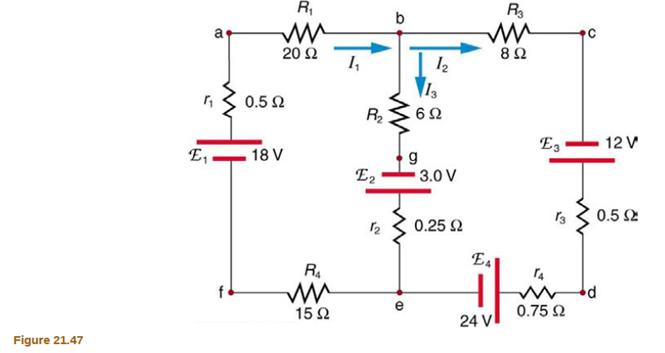Chapter 21, Problem 22CQ

Chapter
Section
Textbook Problem

# Apply the loop rule to loop afedcba in Figure 21.47.To determine

The equation we get after applying the loop rule to loop afedcba.

Explanation

Given:

Formula Used:

According to Kirchhoff's Voltage law

ΔV=0

Calculation:

Using Kirchhoff's Voltage law in loop afedcba, we get

I1r1+E1I1R4E4I2r4I2r3+E3I2R3I1R1=0

Inserting the values

(0

### Still sussing out bartleby?

Check out a sample textbook solution.

See a sample solution

#### The Solution to Your Study Problems

Bartleby provides explanations to thousands of textbook problems written by our experts, many with advanced degrees!

Get Started

## Additional Science Solutions

#### Find more solutions based on key concepts